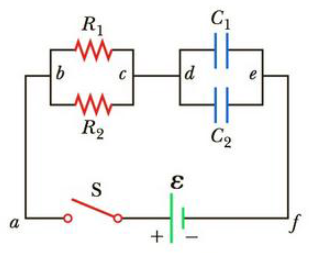Chapter 18, Problem 62AP

Chapter
Section
Textbook Problem

The circuit in Figure P18.62 contains two resistors, R1 = 2.0 kΩ and R2 = 3.0 kΩ, and two capacitors, C1 = 2.0 μF and C2 = 3.0 μF, connected to a battery with emf ε = 120 V. If there are no charges on the capacitors before switch S is dosed, determine the charges q1 and q2 on capacitors C1 and C2, respectively, as functions of time, after the switch is closed. Hint: First reconstruct the circuit so that it becomes a simple RC circuit containing a single resistor and single capacitor in series, connected to the battery, and then determine the total charge q stored in the circuit.Figure P18.62

To determine
The ammeter and voltmeter readings of the circuit.

Explanation

Given Info:

Explanation:

The circuit is,

The potential at the point d is,

Vd=6.0V

The potential at the point e is,

Ve=4.50V

In the above figure, all the points mentioned as a are equal potentials and all the points named as c are also equal potentials.

In the path ecd,

Formula to calculate the voltmeter reading is,

ΔV=VdVe

• Vd is the voltage at d
• Ve is the voltage at e

Substitute 6.0V for Vd and 4.50V for Ve to find the voltmeter reading,

ΔV=6.0V4.50V=1.50V

Thus, the voltmeter reading is 1.50V .

The circuit is,

Apply Kirchhoff’s loop rule in the loop abcfa in the above figure,

(6.0Ω)I+(6.0V)I3=0

Re-write the above expression,

I3=I (I)

Apply Kirchhoff’s loop rule in the loop abcda in the above figure,

(6.0Ω)I+(6

Still sussing out bartleby?

Check out a sample textbook solution.

See a sample solution

The Solution to Your Study Problems

Bartleby provides explanations to thousands of textbook problems written by our experts, many with advanced degrees!

Get Started